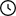• RU
•Waiting For Moderation: 7

# Heat exchanger calculation

• Size: 151 KB

## Description

3. Heat exchanger calculation 3.1 Heat exchanger heat balance calculation The heat balance equation in general form can be written as equations: QUOTE (3.1.1) QUOTE (3.1.2) QUOTE (3.1.3) Then: Q1 = G1 * Cp (t1-t2) (3.1.4) Q2 = X * Cp (t4-t3) (3.1.5) where kg of coolant, G1, X; Cp - average specific heat capacity, J/( kg· ° С); t1, t2- initial and final temperature of hot coolant; t3, t4 is the initial and final temperature of the cold feed. G1 QUOTE Cp (t1-t2) = X-Cp (t4-t3) (3.1.6) We determine the cold coolant flow rate: 2030 * 1039 * ((-70) - (-15)) = X * 1013 ((-135) -12) (3.1.7) X = 779 = G2 Cooling heat load from 12 to -135 ° C: Q = Q1 = Q2 (3.1.8) Q1 = 779 * 1013 * ((-135) - (12) kJ/h Q2 = 1160016 kJ/h The average temperature head Δtcp in the heat exchanger is determined by the Grossf formula: Δtcp = Δtmax - Δtmin/[ 23 1LgΔtmax ]/Δtmin (3.1.9) Δtmax = (-70) - (15) = -85 ° C Δtmin = 12- (-135) = -147 ° C Δtcp = 32 ° C 3.2 Heat exchanger surface calculation The heat exchanger surface value is determined from the main heat transfer equation: Q = K * Δtcp * F (3.2.1) where Q- heat load, kJ; K- heat transfer coefficient = 40 W/m2 K ; F-surface of heat exchange, m2. Fop = Q/K * Δtcp, (3.2.2) Fop = 1160016/40 * 112.9 = 42.6 m2 According to GOST 15118-79, we choose a heat exchanger with a close surface equal to 52 m2, the diameter of the casing is 400 mm single-way with a pipe length of 6 m, the number of pipes 111 pcs; dTp = 25x2, mm; STp = 1,7 QUOTE 102; Sm.tp = 3 QUOTE 102,m2 .  3.3 Refined heat exchanger calculation Heat transfer coefficient is calculated [12, 13]: QUOTE (3.3.1) where a1 a2 are heat transfer coefficients from hot and cold coolant side, W/m2 QUOTE K; QUOTE - wall thickness, equal to 0.002, m ; t- pipe metal thermal conductivity coefficient, W/m2 QUOTE K equal to 46.5 W/m2 QUOTE K . Determine the speed (W, m/s) in the pipe and tube space using the formula QUOTE (3.3.2) where αsr is the average density of raw materials, 860, kg/m3 ; f1 - cross-sectional area of all pipes in one direction of the apparatus, f1 = 0,034 m2 . We calculate the Reynolds criterion: QUOTE, (3.3.3) QUOTE, (3.3.4) where dB, dH - internal and external diameter of pipes; Vcp- kinematic viscosity Vcp = 0,761-10-6, m2/s.  Laminar mode We calculate the Nusselt criterion for tube and tube space: Nu1 = 0,008 QUOTE 0.9 Pr0.43 (3.3.5) Nu2 = 0.2 QUOTE 0.6 Pr0.33 (3.3.6) where Pr- Prandtel criterion, Pr = 5.1. Nu1 = 0.008 QUOTE 365,910.9 QUOTE 5,10.43 = 3.26 Nu2 = 0.2 QUOTE 262,810.6 QUOTE 5,10.33 = 9.68 Heat transfer coefficient from cold and hot coolant is calculated using the formula QUOTE (3.3.7) QUOTE (3.3.8), where a coefficient of heat conductivity of raw materials is 2.45,? Then the heat transfer coefficient is equal to: Required surface: Safety factor is: ∆ = (47.7-42.6) * 100/42.6 = 11.97% According to GOST 15118-79, we choose a heat exchanger with a surface of 57 m2 . 3.4 Hydraulic calculation of the heat exchanger For the transition through the heat exchanger, the heat carriers must be supplied under some excessive pressure to overcome the hydraulic resistance of the QUOTE apparatus (3.4.1) where γ is the friction coefficient; r - number of moves; d - diameter of pipes, mm; QUOTE - the sum of local resistance factors; W is the mass velocity of the medium, kg/m2; p - medium density, kg/m. Local resistance factor takes into account input, output of flows (1.5 QUOTE 2) = 3, rotation between moves (2.5), QUOTE = 5.5:  W = G/S (3.4.2) where G is hot coolant flow rate, G = 1213.2 kg/h; S-section for raw material transition. S = Pd2n/4r (3.4.3) W = 1213,2/0,0785 = 154.5 m/s Pressure loss in pipe space: Annulus resistance: W = 15000/36000uetre where Urethra - cross-sectional area in annulus, m3: Inter - = I/4 (D2 * n * d2H) (3.4.4) Inter = 3.14/4 (12 QUOTE 373 QUOTE 0,0252) = 0,575 m W = 15000 QUOTE 1,022/36000 QUOTE 0,575 = 0,740 kg/m2 QUOTE h Local resistance: input and output = 3, rotation through partition in annulus 4 QUOTE 1.5 = 6. Therefore QUOTE MPa Calculated overpressure does not exceed 1.47 MPa 3.5 Calculation of diameters of heat exchanger nozzles QUOTE (3.5.1) where V is volume flow rate of cold coolant, m3/s; W - cold coolant flow rate, m/s  V = W * SMT = 15.4 QUOTE 7.85 QUOTE 10-2 = 1.2 m3/s (3.4.6) D = 0.31 m As per GOST 9617-76 we accept D = 0.30 m . Similarly, hot coolant nozzles are calculated, D = 0.2 m  is taken.

## Project's Content

Name Sizechertezh_teploobmennika.dwg151 KB